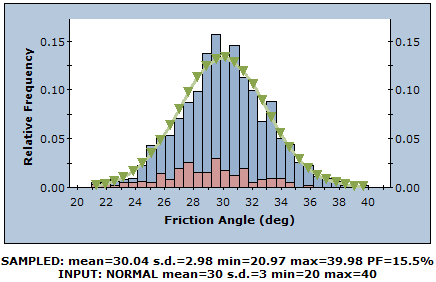Join one of our upcoming courses taking place around the world! Explore now

# Sampling

The Sampling options in the Project Settings dialog allow you to choose:

• Sampling Method
• Number of Samples
• Design Factor of Safety

for a Probabilistic analysis in RocPlane.

## Sampling Method

The Sampling Method determines how the statistical input distributions for the random variables you have defined for a Probabilistic analysis will be sampled. Two Sampling Methods are available in RocPlane:

• Monte Carlo
• Latin Hypercube

NOTE: For both Sampling Methods, sequences of random numbers are utilized to generate the random samples. The generation of random numbers in RocPlane is controlled by the Random Numbers option in the Project Settings dialog.

### MONTE CARLO METHOD

The Monte Carlo sampling technique uses random numbers to sample from the input data probability distributions. Monte Carlo techniques are commonly applied to a wide variety of problems involving random behavior in geotechnical engineering.Monte Carlo sampling of Normal distribution (1000 samples)

### LATIN HYPERCUBE METHOD

The Latin Hypercube sampling technique gives comparable results to the Monte Carlo technique, but with fewer samples [Iman et.al. (1980), Startzman et.al. (1985)]. The method is based upon "stratified" sampling with random selection within each stratum. This results in a smoother sampling of the probability distributions. Typically, an analysis using 1000 samples obtained by the Latin Hypercube technique will produce comparable results to an analysis of 5000 samples using the Monte Carlo method [Hoek et.al. (1995)].Latin Hypercube sampling of Normal distribution (1000 samples)

## Number of Samples

This is the Number of Samples that are generated for each random variable for a Probabilistic Analysis. For example, if Number of Samples = 1000, 1000 values of each input data random variable (e.g., Friction Angle) are generated according to the Sampling Method and Statistical Distribution for each random variable. The analysis will then run 1000 times and a Factor of Safety calculated for each set of input data samples. This results in a distribution of Safety Factors from which the Probability of Failure is calculated.

## Design Factor of Safety

The Design Factor of Safety is applicable to a Probabilistic analysis and is used in the determination of Probability of Failure (i.e., PF = probability of Calculated FS < Design FS) and Number of Failed Wedges. The default value is 1.

NOTE: The Design Factor of Safety does NOT influence the calculation of the Factor of Safety. It is only used as a failure threshold for post-processing of Probabilistic analysis results.